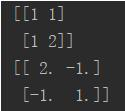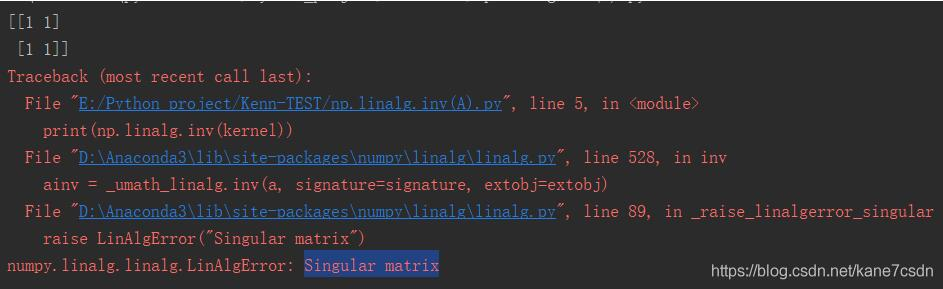### python求逆矩阵的方法,Python 如何求矩阵的逆

import numpy as np

kernel = np.array([1, 1, 1, 2]).reshape((2, 2))

print(kernel)

print(np.linalg.inv(kernel))pinv(A)具有inv(A)的部分特性，但不与inv(A)完全等同。

1.矩阵求逆

import numpy as np

a = np.array([[1, 2], [3, 4]]) # 初始化一个非奇异矩阵(数组)

print(np.linalg.inv(a)) # 对应于MATLAB中 inv() 函数

# 矩阵对象可以通过 .I 求逆,但必须先使用matirx转化

A = np.matrix(a)

print(A.I)

2.矩阵求伪逆

import numpy as np

# 定义一个奇异阵 A

A = np.zeros((4, 4))

A[0, -1] = 1

A[-1, 0] = -1

A = np.matrix(A)

print(A)

# print(A.I) 将报错，矩阵 A 为奇异矩阵，不可逆

print(np.linalg.pinv(A)) # 求矩阵 A 的伪逆(广义逆矩阵)，对应于MATLAB中 pinv() 函数